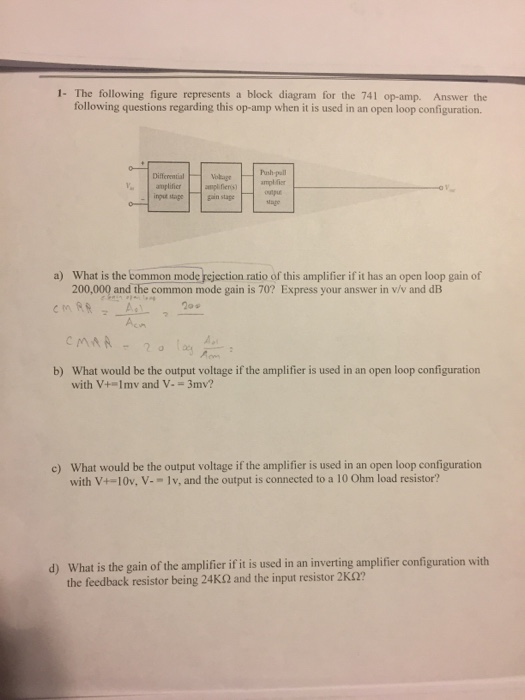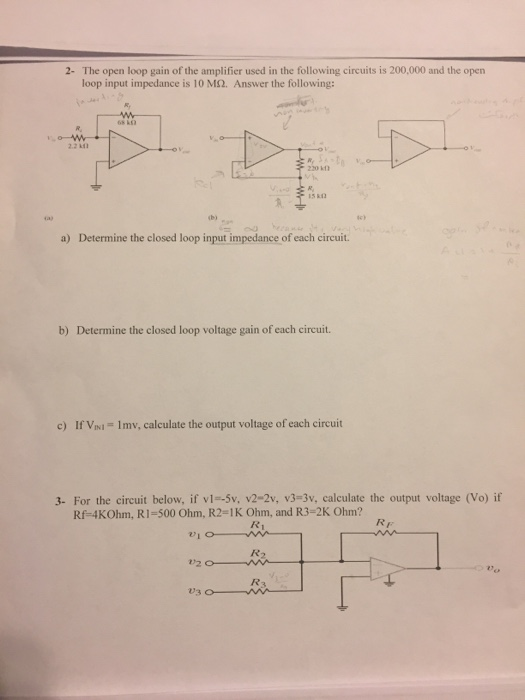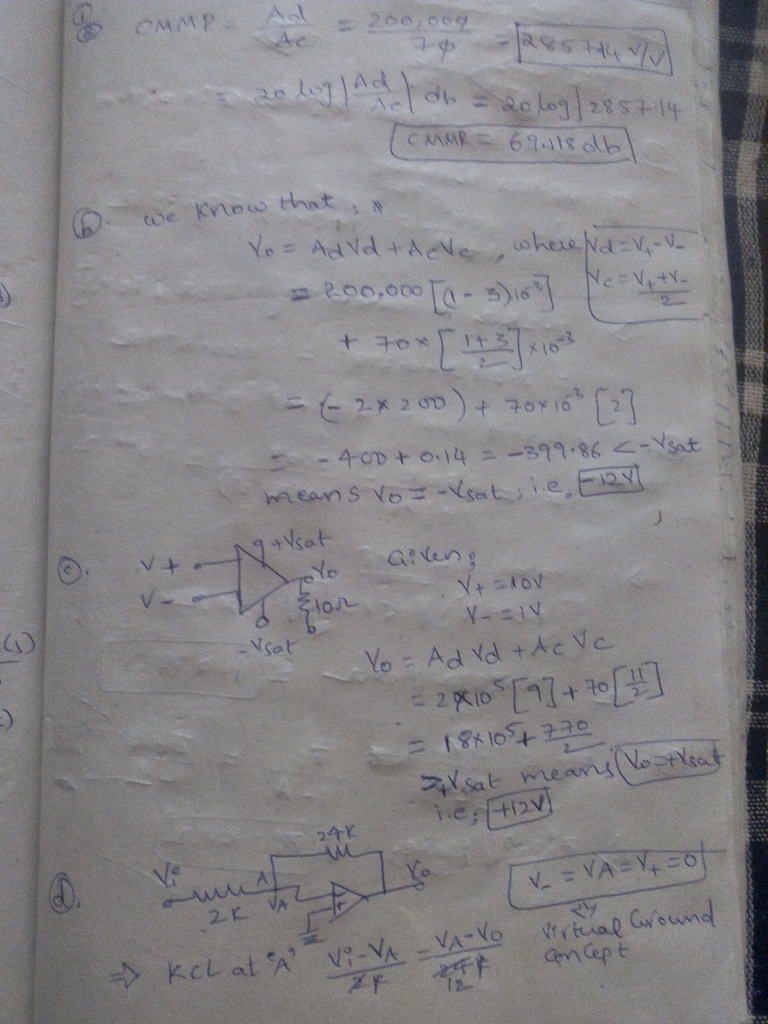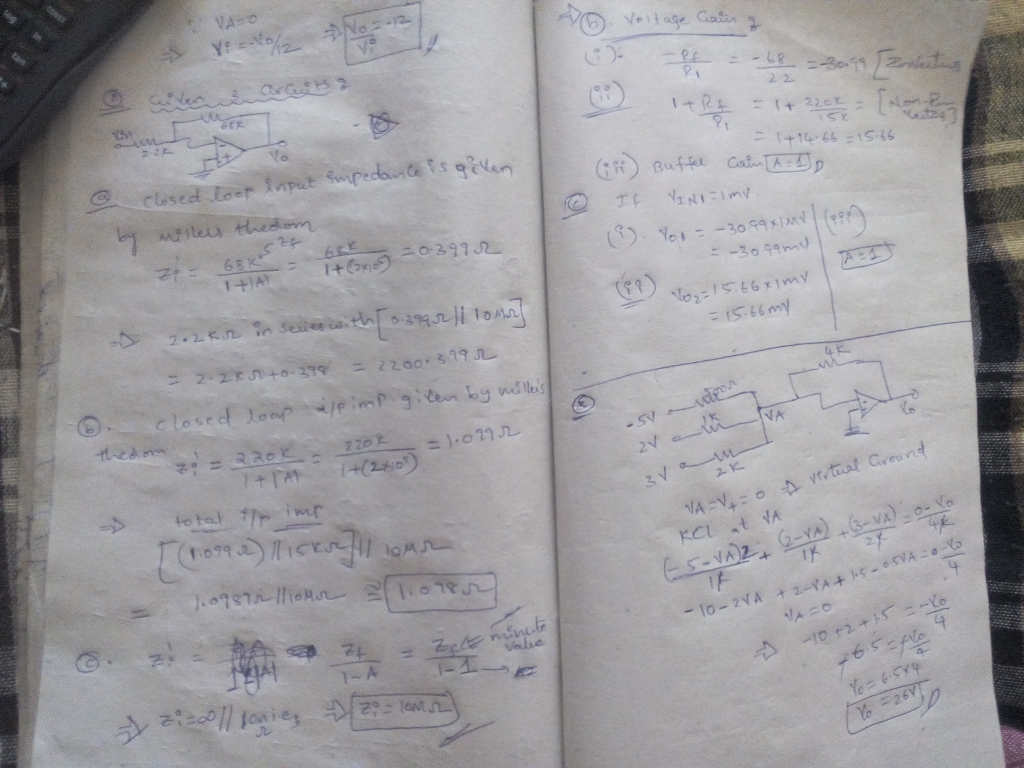# Homework Solution: 1- The following figure represents a block diagram for the 741 op-amp. Answer the following questions regarding this op-amp…

I need answer for all questions.1- The following figure represents a block diagram for the 741 op-amp. Answer the following questions regarding this op-amp when it is used in an open loop configuration. DifferentialVobage amplfier ngud stagenstape lage a) What is the common mode rejection ratio of this amplifier if it has an open loop gain of 200,000 and the common mode gain is 70? Express your answer in v/v and dB 20s What would be the output voltage if the amplifier is used in an open loop configuration with V+=1 mv and V-= 3mv? b) What would be the output voltage if the amplifier is used in an open loop configuration with V+-10v, V--lv, and the output is connected to a lo ohm load resistor? e) What is the gain of the amplifier if it is used in an inverting amplifier configuration with the feedback resistor being 24K02 and the input resistor 2K0? d)